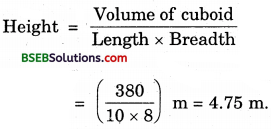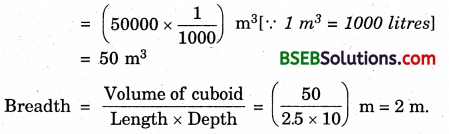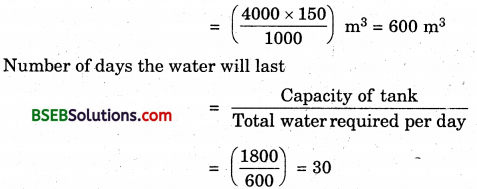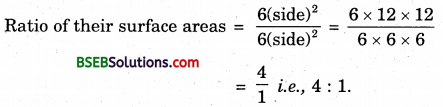# HSSlive: Plus One & Plus Two Notes & Solutions for Kerala State Board

## BSEB Class 9 Maths Chapter 13 Surface Areas and Volumes Ex 13.5 Textbook Solutions PDF: Download Bihar Board STD 9th Maths Chapter 13 Surface Areas and Volumes Ex 13.5 Book AnswersBSEB Class 9 Maths Chapter 13 Surface Areas and Volumes Ex 13.5 Textbook Solutions PDF: Download Bihar Board STD 9th Maths Chapter 13 Surface Areas and Volumes Ex 13.5 Book Answers

BSEB Class 9th Maths Chapter 13 Surface Areas and Volumes Ex 13.5 Textbooks Solutions and answers for students are now available in pdf format. Bihar Board Class 9th Maths Chapter 13 Surface Areas and Volumes Ex 13.5 Book answers and solutions are one of the most important study materials for any student. The Bihar Board Class 9th Maths Chapter 13 Surface Areas and Volumes Ex 13.5 books are published by the Bihar Board Publishers. These Bihar Board Class 9th Maths Chapter 13 Surface Areas and Volumes Ex 13.5 textbooks are prepared by a group of expert faculty members. Students can download these BSEB STD 9th Maths Chapter 13 Surface Areas and Volumes Ex 13.5 book solutions pdf online from this page.

## Bihar Board Class 9th Maths Chapter 13 Surface Areas and Volumes Ex 13.5 Books Solutions

 Board BSEB Materials Textbook Solutions/Guide Format DOC/PDF Class 9th Subject Maths Chapter 13 Surface Areas and Volumes Ex 13.5 Chapters All Provider Hsslive

## How to download Bihar Board Class 9th Maths Chapter 13 Surface Areas and Volumes Ex 13.5 Textbook Solutions Answers PDF Online?

2. Click on the Bihar Board Class 9th Maths Chapter 13 Surface Areas and Volumes Ex 13.5 Answers.
3. Look for your Bihar Board STD 9th Maths Chapter 13 Surface Areas and Volumes Ex 13.5 Textbooks PDF.
4. Now download or read the Bihar Board Class 9th Maths Chapter 13 Surface Areas and Volumes Ex 13.5 Textbook Solutions for PDF Free.

## BSEB Class 9th Maths Chapter 13 Surface Areas and Volumes Ex 13.5 Textbooks Solutions with Answer PDF Download

Find below the list of all BSEB Class 9th Maths Chapter 13 Surface Areas and Volumes Ex 13.5 Textbook Solutions for PDF’s for you to download and prepare for the upcoming exams:

## BSEB Bihar Board Class 9th Maths Solutions Chapter 13 Surface Areas and Volumes Ex 13.5

Question 1.
A matchbox measures 4 cm x 2.5 cm x 1.5 cm. What will be the volume of a packet containing 12 such boxes?
Solution:
Here l = 4 cm, b = 2.5 cm and h = 1.5 cm.
∴Volume of one matchbox = l x b x h cm³
= (4 x 2.5 x 1.5) cm³ = 15 cm³
∴ Volume of a packet containing 12 such boxes
= (12 x 15) cm³ = 180 cm³

Question 2.
A cuboidal water tank is 6 m long, 5 m wide and 4.5 m deep. How many litres of water can it hold? (1 m³ = 1000 l)
Solution:
Here l = 6m, 6 = 5m and h = 4.5 m
∴ Volume of the tank = Ibh m³
= (6 x 5 x 4.5) m³ = 135 m³
∴ The tank can hold = 135 x 1000 litres
= 135000 litres of water. [∵ 1 m³ = 1000 litres]

Question 3.
A cuboidal vessel is 10 m long and 8 m wide. How high must it be made to hold 380 cubic metres of a liquid?
Solution:
Here, length = 10 m, breadth = 8 m and volume = 380 m³Question 4.
Find the cost of digging a cuboidal pit 8 m long, 6 m broad and 3 m deep at the rate of Rs 30 per m³.
Solution:
Here, l = 8 m, b = 6 m and h = 3 m
Volume of the pit = lbh m³
= (8 x 6 x 3) m³ = 144 m³
Rate of digging is Rs 30-per m³
∴ Cost of digging the pit = Rs (144 x 30) = Rs 4320.

Question 5.
The capacity of a cuboidal tank is 50000 litres of water. Find the breadth of the tank, if its length and depth are respectively 2.5 m and 10 m.
Solution:
Here, length = 2.5 m, depth = 10 m
and volume = 50000 litresQuestion 6.
A village, having a population of 4000, requires 150 litres of water per head per day. It has a tank measuring 20 m x 15 m x 6 m. For how many days will the water of this tank last?
Solution:
Here, l = 20 m, 6 = 15 m and h = 6 m.
∴ Capacity of the tank = lbh m³
= (20 x 15 x 6) m³ = 1800 m³
Water requirement per person per day
= 150 litres
Water required for 4000 person per day
= (4000 x 150) l
= (4000×1501000) m³ = 600 m³
Number of days the water will lastThus, the water will last for 30 days.

Question 7.
A godown measures 60 m x 25 m x 10 m. Find the maximum number of wooden crates each measuring 1.5 m x 1.25 m x 0.5 m that can be stored in the godown.
Solution:
Volume of the godown = (60 x 25 x 10) m³ = 15000 m³
Volume of 1 crates = (1.5 x 1.25 x 0.5) m³ = 0.9375 m³
Number of crates that can be stored in the godownQuestion 8.
A solid cube of side 12 cm is cut into eight cubes of equal volume. What will be the side of the new cube? Also, find the ratio between their surface areas.
Solution:
Let V1 = Volume of the cube of edge 12 cm = (12 x 12 x 12) cm³
V1 = Volume of the cube cut out of the first one
= 18 x V1 = (18 x 12 x 12 x 12) cm³
= (6 x 6 x 6) cm³
∴ Side of the new cube = 6 cmQuestion 9.
A river 3 m deep and 40 m wide is flowing at the rate of 2 km per hour. How much water will fall into the sea in a minute?
Solution:
Since the water flows at the rate of 2 km per hour, the water from 2 km of river flows into the sea in one hour.
∴ The volume of water flowing into the sea in one hour
= Volume of the cuboid
= l x b x h m³
= (2000 x 40 x 3) m³
∴ The volume of water flowing into the sea in one minute
= (2000×40×360) m³
= 4000 m³.

## Bihar Board Class 9th Maths Chapter 13 Surface Areas and Volumes Ex 13.5 Textbooks for Exam Preparations

Bihar Board Class 9th Maths Chapter 13 Surface Areas and Volumes Ex 13.5 Textbook Solutions can be of great help in your Bihar Board Class 9th Maths Chapter 13 Surface Areas and Volumes Ex 13.5 exam preparation. The BSEB STD 9th Maths Chapter 13 Surface Areas and Volumes Ex 13.5 Textbooks study material, used with the English medium textbooks, can help you complete the entire Class 9th Maths Chapter 13 Surface Areas and Volumes Ex 13.5 Books State Board syllabus with maximum efficiency.

## FAQs Regarding Bihar Board Class 9th Maths Chapter 13 Surface Areas and Volumes Ex 13.5 Textbook Solutions

#### How to get BSEB Class 9th Maths Chapter 13 Surface Areas and Volumes Ex 13.5 Textbook Answers??

Students can download the Bihar Board Class 9 Maths Chapter 13 Surface Areas and Volumes Ex 13.5 Answers PDF from the links provided above.

#### Can we get a Bihar Board Book PDF for all Classes?

Yes you can get Bihar Board Text Book PDF for all classes using the links provided in the above article.

## Important Terms

Bihar Board Class 9th Maths Chapter 13 Surface Areas and Volumes Ex 13.5, BSEB Class 9th Maths Chapter 13 Surface Areas and Volumes Ex 13.5 Textbooks, Bihar Board Class 9th Maths Chapter 13 Surface Areas and Volumes Ex 13.5, Bihar Board Class 9th Maths Chapter 13 Surface Areas and Volumes Ex 13.5 Textbook solutions, BSEB Class 9th Maths Chapter 13 Surface Areas and Volumes Ex 13.5 Textbooks Solutions, Bihar Board STD 9th Maths Chapter 13 Surface Areas and Volumes Ex 13.5, BSEB STD 9th Maths Chapter 13 Surface Areas and Volumes Ex 13.5 Textbooks, Bihar Board STD 9th Maths Chapter 13 Surface Areas and Volumes Ex 13.5, Bihar Board STD 9th Maths Chapter 13 Surface Areas and Volumes Ex 13.5 Textbook solutions, BSEB STD 9th Maths Chapter 13 Surface Areas and Volumes Ex 13.5 Textbooks Solutions,
Share: